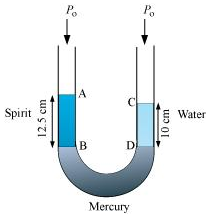## Pages

### Mechanical Properties of Fluids NCERT Solutions Class 11 Physics - Solved Exercise Question 10.9

Question 10.9:
A U-tube contains water and methylated spirit separated by mercury. The mercury columns in the two arms are in level with 10.0 cm of water in one arm and 12.5 cm of spirit in the other. What is the specific gravity of spirit?
Solution:
The given system of water, mercury, and methylated spirit is shown as follows:Height of the spirit column, h1 = 12.5 cm = 0.125 m
Height of the water column, h2 = 10 cm = 0.1 m
P0 = Atmospheric pressure
ρ1 = Density of spirit
ρ2 = Density of water
Pressure at point B = P0 + ρ1h1g
Pressure at point D = P0 + ρ2h2g
Pressure at points B and D is the same.
P0 + ρ1h1g  =  P0 + ρ2h2g
ρ1 / ρ2  =  h2 / h1
= 10 / 12.5  =  0.8
Therefore, the specific gravity of spirit is 0.8.